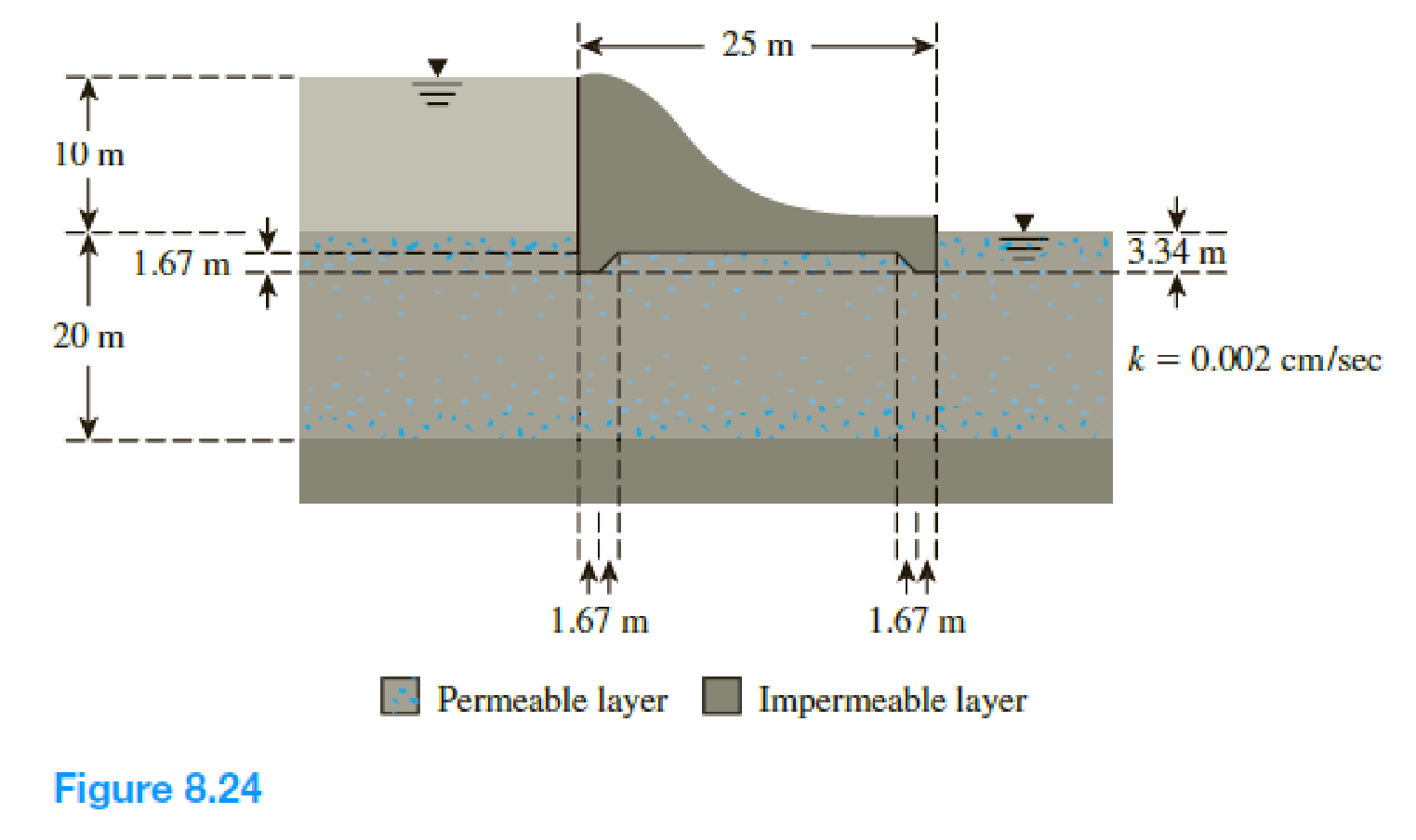Chapter 8, Problem 8.5PPrinciples of Geotechnical Enginee...

9th Edition
Braja M. Das + 1 other
ISBN: 9781305970939

Solutions

Chapter
SectionPrinciples of Geotechnical Enginee...

9th Edition
Braja M. Das + 1 other
ISBN: 9781305970939
Textbook Problem

Refer to Problem 8.4. Using the flow net drawn, calculate the hydraulic uplift force at the base of the hydraulic structure per meter length (measured along the axis of the structure).  8.24 For the hydraulic structure shown in Figure 8.24, draw a flow net for flow through the permeable layer and calculate the seepage loss in m3/day/m.To determine

Find the hydraulic uplift force at the base of the hydraulic structure per meter length.

Explanation

Given information:

The hydraulic conductivity of the permeable soil layer k is 0.002cm/sec.

The head difference between the upstream and downstream H is 10 m.

The height of the water level H2 is 3.34 m.

The depth of permeable layer up to the tip of the hydraulic structure D is 1.67 m.

The depth of permeable layer D1 is 20 m.

Calculation:

Draw the free body diagram of the flow net for the given values as in Figure 1.

Determine the head loss for each drop using the relation.

ΔH=HNd

Here, Nd is the number of potential drop.

Refer Figure 1.

The number of potential drop Nd is 12.

Substitute 10 m for H and 12 for Nd.

ΔH=1012=0.83m

Determine the pressure head at D using the relation.

(ph)D=(H+H1)flowdis×ΔH

Here, flow dis is the flow net distance.

Substitute 10 m for H, 3.34 m for H1, 2 m for flow dis, and 0.833 for ΔH.

(ph)D=(10+3.34)2×0.833=11.67m

Determine the pressure head at E using the relation.

(ph)E=(H+H1)flowdis×ΔH

Substitute 10 m for H, 3.34 m for H1, 3 m for flow dis, and 0.833 for ΔH.

(ρh)E=(10+3.34)3×0.833=10.84m

Determine the pressure head at F using the relation.

(ph)F=(H+D)flowdis×ΔH

Substitute 10 m for H, 1.67 m for D, 3.5 m for flow dis, and 0.833 for ΔH.

(ph)F=(10+1.67)3.5×0.833=8.75m

Determine the pressure head at G using the relation.

(ph)G=(H+D)flowdis×ΔH

Substitute 10 m for H, 1.67 m for D, 8.5 m for flow dis, and 0.833 for ΔH.

(ph)G=(10+1.67)8.5×0.833=4.586m

Determine the pressure head at H using the relation.

(ph)H=(H+H1)flowdis×ΔH

Substitute 10 m for H, 3.34 m for H1, 9 m for flow dis, and 0.833 for ΔH.

(ph)H=(10+3.34)9×0.833=5.84m

Determine the pressure head at I using the relation.

(ph)I=(H+H1)flowdis×ΔH

Substitute 10 m for H, 3.34 m for H1, 10 m for flow dis, and 0.833 for ΔH.

(ph)I=(10+3.34)10×0.833=5.0m

Determine the hydraulic uplift force at the base of the hydraulic structure per meter length using the relation

Still sussing out bartleby?

Check out a sample textbook solution.

See a sample solution

The Solution to Your Study Problems

Bartleby provides explanations to thousands of textbook problems written by our experts, many with advanced degrees!

Get Started

What do nuclear engineers do?

Engineering Fundamentals: An Introduction to Engineering (MindTap Course List)

Identify several BI tools.

Fundamentals of Information Systems

Describe the purpose of an ECT signal on an EFI system.

Automotive Technology: A Systems Approach (MindTap Course List)

What method is used to harden low-carbon steels?

Precision Machining Technology (MindTap Course List)

What is a sacrificial host? What is a bastion host?

Principles of Information Security (MindTap Course List)

What is the difference between a detail report, a summary report, and an exception report?

Systems Analysis and Design (Shelly Cashman Series) (MindTap Course List)

What four welding processes are covered in the SENSE program?

Welding: Principles and Applications (MindTap Course List)

What types of businesses use a dye-sublimation printer?

Enhanced Discovering Computers 2017 (Shelly Cashman Series) (MindTap Course List)

What is the fundamental distinction between a MAN and a WAN?

Network+ Guide to Networks (MindTap Course List)